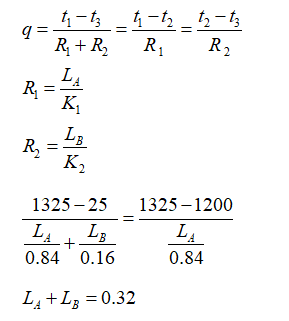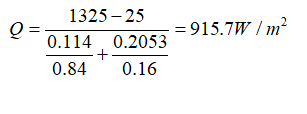# A reactor's wall 320mm thick, is made up of an inner layer of fire brick (k = 0.84W/m°C) covered with a layer of insulation (k = 0.16W/m°C). The reactor operates at a temperature of 1325°C at the atmospheric temperature of 25°C. i. Calculate the thickness of brick and insulation. ii. Heat loss per unit area presuming that the insulating material has a maximum temperature of 1200°C.

Question-AnswerCategory: Heat And Mass TransferA reactor's wall 320mm thick, is made up of an inner layer of fire brick (k = 0.84W/m°C) covered with a layer of insulation (k = 0.16W/m°C). The reactor operates at a temperature of 1325°C at the atmospheric temperature of 25°C. i. Calculate the thickness of brick and insulation. ii. Heat loss per unit area presuming that the insulating material has a maximum temperature of 1200°C.

A reactor’s wall 320mm thick, is made up of an inner layer of fire brick (k = 0.84W/m°C) covered with a layer of insulation (k = 0.16W/m°C). The reactor operates at a temperature of 1325°C at the atmospheric temperature of 25°C.

i. Calculate the thickness of brick and insulation.

ii. Heat loss per unit area presuming that the insulating material has a maximum temperature of 1200°C.

Given Data
The thickness of the reactor wall, t=320 mm
The thermal conductivity, K1= 0.84W/m°C  k2 = 0.16W/m°C
The reactor temperature Ti=1325°C
The atmospheric temperature, T0=25°C
The maximum temperature of insulating material, Tmax=1200°CSubstitute in above equations we get
LA=114.7mm  and LB=205.3mm

Heat loss per unit area,